Modelling object-oriented software – an introduction

Start this free course now. Just create an account and sign in. Enrol and complete the course for a free statement of participation or digital badge if available.

Free course

# 4.3 Multiplicity

When discussing the associations for the DVD Library System, we noted that a requirements document frequently provides information about the number of links that are possible between objects of particular classes. For example, the requirements stated that ‘no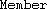can have more than six DVDs on loan at once’. For the sake of simplicity we will assume that a loan involves a single DVD. Therefore, in terms of the model, this means that noobject may have links to more than six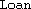objects at any one time (though it may have fewer such links).

To illustrate this, the object diagram in Figure 13 represents a situation where there are three members of the library. At the point in time depicted in the diagram,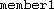has three DVDs on loan,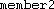has no current loans, and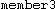has one DVD on loan.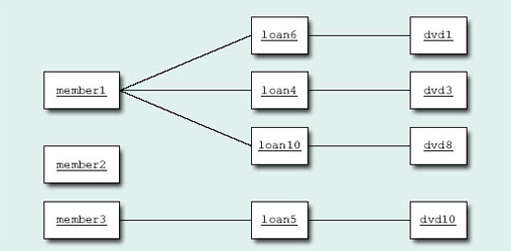Figure 13 Object diagram showing isToand isOf links

The number of instances of a classthat can be linked by a particular association to a single instance of the classat the other end of the association is known as the multiplicity of the association at theend. For example, suppose that each student on a particular course must have a single tutor, and that each tutor must be assigned to 20 students. The multiplicities of a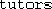association between the classes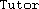and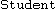would be shown as in Figure 14.Figure 14 Multiplicities for aassociation betweenand.

Figure 14 tells us that eachobject must be linked to exactly oneobject and that eachobject must be linked to 20objects.

The multiplicity may be a single figure or a range. For example, a member of the DVD Library may, at any point in time, have any number of loans between 0 and 6. That is, the multiplicity of the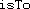association at theend is ‘zero to six’ and would be indicated on the class diagram by writing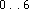on theassociation next to theclass. Although a member may have a number of loans at a particular time, each loan will be linked to only a single member, so the multiplicity ofat theend is(sometimes expressed as ‘exactly one’). Figure 15 shows the class diagram for the DVD Library System with the multiplicities foradded.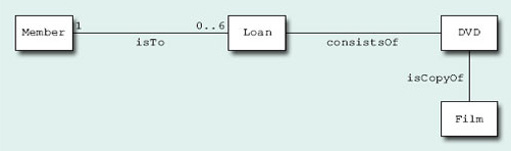Figure 15 Class diagram for the DVD Library System showing multiplicities for theassociation

Now consider the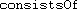association. A particularobject will always be linked to a single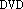object. The multiplicity ofis thereforeat theend. However, a DVD may or may not be on loan, so a particularobject is linked to zero or oneobjects and the multiplicity ofat theend is therefore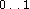(described as ‘zero or one’).

## Self-assessment question 3

What is the multiplicity of the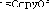association at the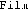end?

A particular DVD is a copy of a single film, so the multiplicity at theend ofis.

What about theend of? The requirements state that the library ‘… may have any number of copies of each film’. In other words, there may be no copies at all, but there is no upper limit. Such a multiplicity is expressed as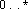(described as ‘zero or more’), where * indicates that there are no upper restrictions on the number. Figure 16 shows the class diagram for the system with all the multiplicities added.

In this context, the * is usually referred to as ‘star’.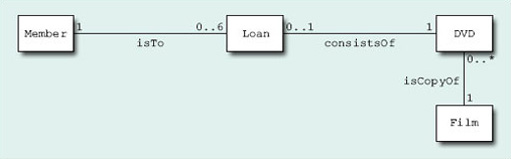Figure 16 Class diagram for the DVD Library System, showing multiplicities for all associations

Multiplicity ranges do not need to start with 0. A statement such as ‘Members of a book club must choose at least two books from the titles on offer each quarter, but may purchase up to six books at the offer price’ would give rise to a multiplicity of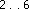(described as ‘two to six’) at the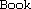end of a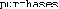association between the classesand. Multiplicities are always written with the smaller number in the range first.

## Self-assessment question 4

Use Figure 17 (below) to answer the following questions.

• (a) How many courses can a student take?

• (b) How many tutors teach each course?

• (c) How many students can enrol for a course?

• (d) How many courses can each tutor teach?Figure 17 Class diagram representing students, courses and tutors

• (a) Between 1 and 4, inclusive (i.e. 1, 2, 3 or 4).

• (b) Exactly 1.

• (c) Zero or more (i.e. any number).

• (d) Between 1 and 3, inclusive (i.e. 1, 2 or 3).

The multiplicities of an association answer important questions about the relationship between the two classes involved. The answers to these questions will play a significant part in later phases of the software development process. Questions which are of particular relevance in this respect are:

• Must an instance of one class be linked to at least one instance of the class at the other end of the association, or is it possible that, at a certain point in time, no such links exist? For example, everyobject in the DVD Library System must be linked to aobject (a multiplicity of 1), whereas aobject may or may not be linked to anyobject at a particular point in time (a multiplicity of).

• May an instance of one class be linked to more than one instance of the class at the other end of the association? For example, aobject is always linked to a singleobject (a multiplicity of 1) at any one time, whereas aobject may be linked to 0, 1 or moreobjects (a multiplicity of).

• Is there an upper limit to the number of possible links that may exist from an object of one class to objects of the class at the other end of the association? For example, aobject may have at most six links toobjects.

## Self-assessment question 5

Using Figure 17 for reference, describe in words the multiplicities at each end of the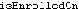and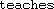associations.has a multiplicity of zero or more at theend and one to four at the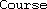end.has a multiplicity of one to three at theend and one (or exactly one) at theend.

M256_1